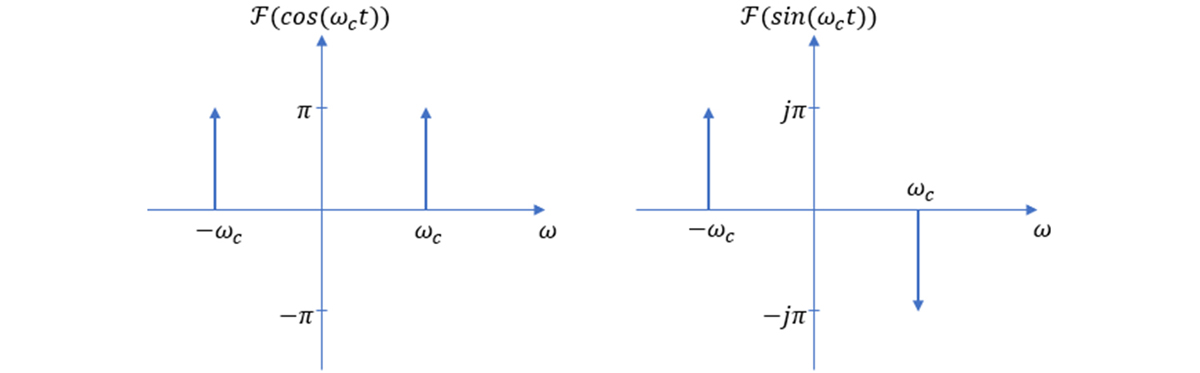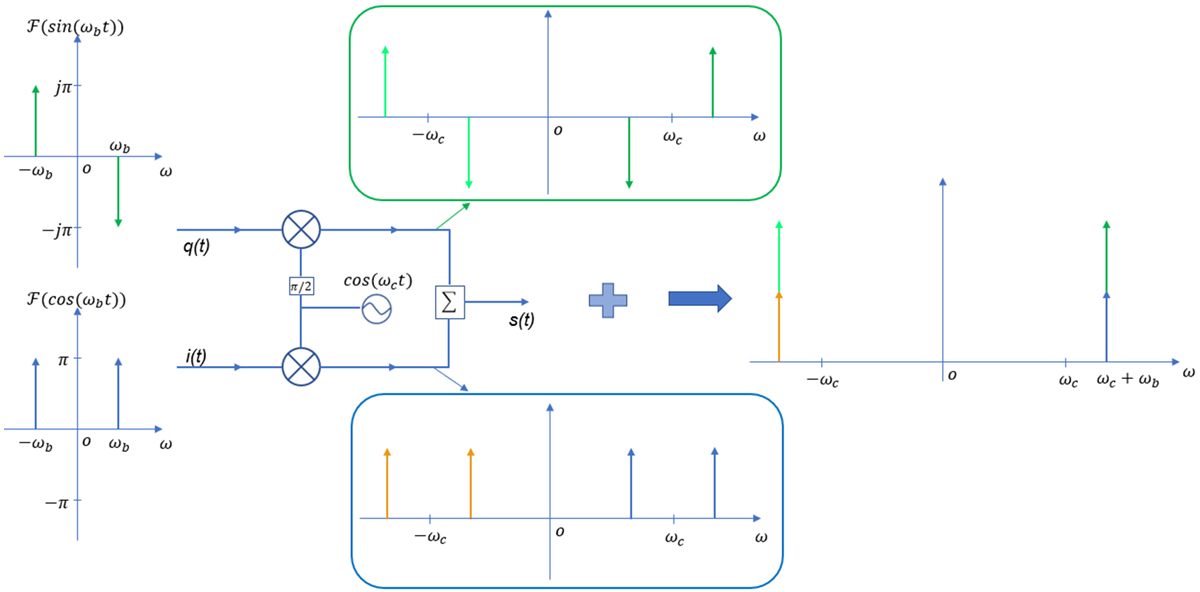# 数字调制系列：IQ调制器特性

2020-06-14 来源：微波射频网作者：Knight 我要评论(0) 字号：

1. IQ 信号幅度平衡性对镜频抑制的影响

IQ信号幅度不平衡(即幅度不同)，要么是输入至调制器的I 和Q 信号的幅度不平衡，要么是调制器具有一定的增益不平衡(即I 和Q 两路的增益不同)，这些都会影响对镜频的抑制能力。

i(t)=Acoswbtq(t)=sinwbt，则经过IQ调制输出的射频信号s(t)

s(t)=Acoswbt· coswct - sinwbt · sinwct

s(t)=0.5(A+1)cos(wc+wb)t + 0.5(A-1)cos(wc-wb)t

A=1时，射频信号中只有上边带(wc+wb)分量；

A=-1时，射频信号中只有下边带(wc-wb)分量；

A≠±1时，射频信号中同时包含上边带(wc+wb)和下边带(wc-wb)两个分量。A≠±1时，射频信号中同时包含上下边带，定义边带抑制比为：20lg│A+1│/│A-1│ (dB).

IQ 调制器两个支路的增益不平衡特性已经无法调整，但是可以在基带侧通过调整I 和Q 两路波形的幅度大小改善镜频抑制。矢量信号发生器VSG及任意波信号发生器AWG均提供了IQ Gain Imbalance调整参数，对其进行微调即可改善镜频抑制。

2. IQ 正交性对镜频抑制的影响

i(t)=cos(wbt+ϕ)q(t)=sinwbt，则IQ 调制器输出的射频信号为

s(t)=cos(wbt+ϕ)· coswct - sinwbt · sinwct

s(t)=0.5(1+cosϕ)·cos(wc+wb)t-0.5sinϕ·sin(wc+wb)t-0.5(1-cosϕ)·cos(wc-wb)t+0.5sinϕ·sin(wc-wb)t

cosθ=a/√( a2+b2)sinθ=b/√( a2+b2)

cosθ1=c/√(c2+b2)sinθ1=b/√(c2+b2)

s(t)=0.707√(1+cosϕ)·cos[(wc+wb)t+θ]+0.707√(1-cosϕ)·cos[(wc-wb)t-θ1]

3. IQ 调制器的载波抑制特性

IQ 调制器除了可以抑制镜频外，在数字调制过程中还可以抑制载波。理论上，只要模拟I 和Q 信号中没有DC 分量，而且IQ 调制器是理想的，那么输出的射频宽带信号中将没有载波。但是实际产生的宽带信号总是具有一定的载波泄露，来源于两部分：(1) IQ 信号中包含一定的DC 分量；(2) IQ 调制器中Mixer 的LO 泄露。

 微波射频行业人士 | 相聚在这里【10大细分领域的微信技术交流群】订阅微信公众号加群管理员为好友